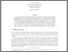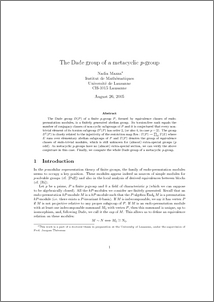# The Dade group of a metacyclic $p$-group.

Mazza, Nadia (2003) The Dade group of a metacyclic $p$-group. Journal of Algebra, 266 (1). pp. 102-111. ISSN 0021-8693Preview
PDF (dade-group-of-a-metacyclic-p-group.pdf)
dade-group-of-a-metacyclic-p-group.pdf

Download (253kB)

## Abstract

The Dade group $D(P)$ of a finite $p$-group $P$, formed by equivalence classes of endo-permutation modules, is a finitely generated abelian group. Its torsion-free rank equals the number of conjugacy classes of non-cyclic subgroups of $P$ and it is conjectured that every non-trivial element of its torsion subgroup $D^t(P)$ has order $2$, (or also $4$, in case $p=2$). The group $D^t(P)$ is closely related to the injectivity of the restriction map $\Res:T(P)\rightarrow\prod_E T(E)$ where $E$ runs over elementary abelian subgroups of $P$ and $T(P)$ denotes the group of equivalence classes of endo-trivial modules, which is still unknown for (almost) extra-special groups ($p$ odd). As metacyclic $p$-groups have no (almost) extra-special section, we can verify the above conjecture in this case. Finally, we compute the whole Dade group of a metacyclic $p$-group.

Item Type: Journal Article Journal of Algebra The final, definitive version of this article has been published in the Journal, Journal of Algebra 266 (1), 2003, © ELSEVIER. /dk/atira/pure/researchoutput/libraryofcongress/qa Faculty of Science and Technology > Mathematics and Statistics 20815 Dr Nadia Mazza 03 Dec 2008 11:19 No Published 19 Aug 2019 00:21 https://eprints.lancs.ac.uk/id/eprint/20815

### Actions (login required)View Item